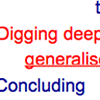# Resources tagged with: Proof by contradiction

Filter by: Content type:
Age range:
Challenge level:

There are 12 NRICH Mathematical resources connected to Proof by contradiction, you may find related items under Thinking Mathematically.### Eyes Down

##### Age 16 to 18Challenge Level

The symbol [ ] means 'the integer part of'. Can the numbers [2x]; 2[x]; [x + 1/2] + [x - 1/2] ever be equal? Can they ever take three different values?### Mastering Mathematics: the Challenge of Generalising and Proof

##### Age 5 to 11

This article for primary teachers discusses how we can help learners generalise and prove, using NRICH tasks as examples.### Impossible Square?

##### Age 16 to 18Challenge Level

Can you make a square from these triangles?### Rarity

##### Age 16 to 18Challenge Level

Show that it is rare for a ratio of ratios to be rational.### An Introduction to Proof by Contradiction

##### Age 14 to 18

An introduction to proof by contradiction, a powerful method of mathematical proof.### The Dangerous Ratio

##### Age 11 to 14

This article for pupils and teachers looks at a number that even the great mathematician, Pythagoras, found terrifying.### Rational Round

##### Age 16 to 18Challenge Level

Show that there are infinitely many rational points on the unit circle and no rational points on the circle x^2+y^2=3.### Proof Sorter - the Square Root of 2 Is Irrational

##### Age 16 to 18Challenge Level

Try this interactivity to familiarise yourself with the proof that the square root of 2 is irrational. Sort the steps of the proof into the correct order.### Staircase

##### Age 16 to 18Challenge Level

Solving the equation x^3 = 3 is easy but what about solving equations with a 'staircase' of powers?### Tetra Inequalities

##### Age 16 to 18Challenge Level

Prove that in every tetrahedron there is a vertex such that the three edges meeting there have lengths which could be the sides of a triangle.### Proximity

##### Age 14 to 16Challenge Level

We are given a regular icosahedron having three red vertices. Show that it has a vertex that has at least two red neighbours.### Be Reasonable

##### Age 16 to 18Challenge Level

Prove that sqrt2, sqrt3 and sqrt5 cannot be terms of ANY arithmetic progression.